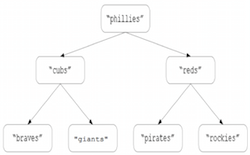### CSC 421: Algorithm Design and Analysis Spring 2016 HW3: Binary Search/Select Trees

In CSC 321, we considered basic implementations of binary trees and (through inheritance) binary search trees. The following classes are variations of the versions from last semester: TreeNode.java, BinaryTree.java, BinarySearchTree.java. The `BinaryTree` class contains an O(N) `size` method, and the `BinarySearchTree` class contains O(N) `select` and `rank` methods. For this assignment, you will modify these classes, trading space for time, so that the `size` operation becomes O(1) and the `select` and `rank` operations become O(log N).

### Part 1: O(1) `size`

Currently, the `size` method uses divide-and-conquer recursion to traverse the tree and count the number of nodes. To avoid the linear cost of this approach, you are to modify `TreeNode` so that in addition to storing the data value and subtrees, each node also stores the sizes of its subtrees. With this size information embedded in each node, it becomes simple to determine the size of any subtree in a tree. For example, suppose `current` referred to a node in a binary tree. if you knew that the left subtree of `current` contained 12 nodes, and the right subtree of `current` contained 14 nodes, then the tree rooted at `current` would contain 12+14+1 = 27 nodes.

Note: all of the `TreeNode` methods should remain O(1).

Once you have made your modifications, reimplement the `size` method in `BinaryTree` so that it utilizes the size information in nodes to determine the tree size in O(1) time.

### Part 2: O(log N) `select`

Currently, the `select` method in `BinarySearchTree` is O(N), since it first converts the tree into a list and then accesses the specified index in that list. Alternatively, a decrease-and-conquer approach can be taken, similar to the approach used in quick-select (as described in lectures). Consider the following binary search tree:Suppose you want the rank-3 value in the tree. Since there are 3 values in the left subtree (corresponding to indices 0-2), the rank-3 value must be at the root. If you wanted the rank-2 value in the tree, it must be in the left subtree (and in fact is the rank-2 value in the left subtree). If you wanted the rank-4 value in the tree, it must be in the right subtree (and in fact is the rank-0 value in the right subtree).

You are to implement the `select` operation using this decrease-and-conquer approach, which does work proportional to the height of the binary search tree. As long as the tree is relatively balanced, the `select` operation will be O(log N).

### Part 3: O(log N) `rank`

Similarly, it is possible to utilize the size information in nodes to implement an O(log N) `BinarySearchTree rank` operation. Recall that the `rank` operation is the inverse of `select` - it finds a specific value in the tree and returns its order rank. For example, the rank of "cubs" in the above binary search tree is 1, whereas the rank of "pirates" is 4.

You are to implement the `rank` method using a decrease-and-conquer approach, so that the amount of work is proportional to the height of the binary search tree.